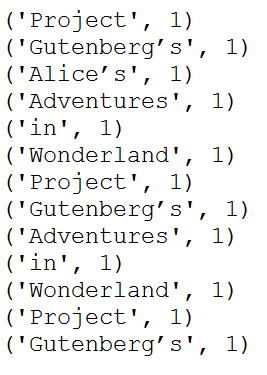# PySpark map() Transformation

PySpark map (`map()`) is an RDD transformation that is used to apply the transformation function (lambda) on every element of RDD/DataFrame and returns a new RDD. In this article, you will learn the syntax and usage of the RDD map() transformation with an example and how to use it with DataFrame.

RDD `map()` transformation is used to apply any complex operations like adding a column, updating a column, transforming the data e.t.c, the output of map transformations would always have the same number of records as input.

• Note1: DataFrame doesn’t have map() transformation to use with DataFrame hence you need to DataFrame to RDD first.
• Note2: If you have a heavy initialization use PySpark mapPartitions() transformation instead of map(), as with mapPartitions() heavy initialization executes only once for each partition instead of every record.

First, let’s create an RDD from the list.

``````
from pyspark.sql import SparkSession
spark = SparkSession.builder.master("local") \
.appName("SparkByExamples.com").getOrCreate()

"in","Wonderland","Project","Gutenberg’s"]

rdd=spark.sparkContext.parallelize(data)
``````

## map() Syntax

``````
map(f, preservesPartitioning=False)
``````

## PySpark map() Example with RDD

In this PySpark `map()` example, we are adding a new element with value 1 for each element, the result of the RDD is `PairRDDFunctions` which contains key-value pairs, word of type String as Key and 1 of type Int as value.

``````
rdd2=rdd.map(lambda x: (x,1))
for element in rdd2.collect():
print(element)
``````

This yields below output.

## PySpark map() Example with DataFrame

PySpark DataFrame doesn’t have `map()` transformation to apply the lambda function, when you wanted to apply the custom transformation, you need to convert the DataFrame to RDD and apply the map() transformation. Let’s use another dataset to explain this.

``````
data = [('James','Smith','M',30),
('Anna','Rose','F',41),
('Robert','Williams','M',62),
]

columns = ["firstname","lastname","gender","salary"]
df = spark.createDataFrame(data=data, schema = columns)
df.show()
+---------+--------+------+------+
|firstname|lastname|gender|salary|
+---------+--------+------+------+
|    James|   Smith|     M|    30|
|     Anna|    Rose|     F|    41|
|   Robert|Williams|     M|    62|
+---------+--------+------+------+
``````
``````
# Refering columns by index.
rdd2=df.rdd.map(lambda x:
(x+","+x,x,x*2)
)
df2=rdd2.toDF(["name","gender","new_salary"]   )
df2.show()
+---------------+------+----------+
|           name|gender|new_salary|
+---------------+------+----------+
|    James,Smith|     M|        60|
|      Anna,Rose|     F|        82|
|Robert,Williams|     M|       124|
+---------------+------+----------+
``````

Note that aboveI have used index to get the column values, alternatively, you can also refer to the DataFrame column names while iterating.

``````
# Referring Column Names
rdd2=df.rdd.map(lambda x:
(x["firstname"]+","+x["lastname"],x["gender"],x["salary"]*2)
)
``````

Another alternative

``````
# Referring Column Names
rdd2=df.rdd.map(lambda x:
(x.firstname+","+x.lastname,x.gender,x.salary*2)
)
``````

You can also create a custom function to perform an operation. Below `func1()` function executes for every DataFrame row from the lambda function.

``````
# By Calling function
def func1(x):
firstName=x.firstname
lastName=x.lastname
name=firstName+","+lastName
gender=x.gender.lower()
salary=x.salary*2
return (name,gender,salary)

rdd2=df.rdd.map(lambda x: func1(x))
``````

## Complete PySpark map() example

Below is complete example of PySpark map() transformation.

``````
from pyspark.sql import SparkSession
spark = SparkSession.builder.appName('SparkByExamples.com').getOrCreate()

data = ["Project",
"Gutenberg’s",
"Alice’s",
"in",
"Wonderland",
"Project",
"Gutenberg’s",
"in",
"Wonderland",
"Project",
"Gutenberg’s"]

rdd=spark.sparkContext.parallelize(data)

rdd2=rdd.map(lambda x: (x,1))
for element in rdd2.collect():
print(element)

data = [('James','Smith','M',30),
('Anna','Rose','F',41),
('Robert','Williams','M',62),
]

columns = ["firstname","lastname","gender","salary"]
df = spark.createDataFrame(data=data, schema = columns)
df.show()

rdd2=df.rdd.map(lambda x:
(x+","+x,x,x*2)
)
df2=rdd2.toDF(["name","gender","new_salary"]   )
df2.show()

#Referring Column Names
rdd2=df.rdd.map(lambda x:
(x["firstname"]+","+x["lastname"],x["gender"],x["salary"]*2)
)

#Referring Column Names
rdd2=df.rdd.map(lambda x:
(x.firstname+","+x.lastname,x.gender,x.salary*2)
)

def func1(x):
firstName=x.firstname
lastName=x.lastname
name=firstName+","+lastName
gender=x.gender.lower()
salary=x.salary*2
return (name,gender,salary)

rdd2=df.rdd.map(lambda x: func1(x))
``````

In conclusion, you have learned how to apply a `map()` transformation on every element of PySpark RDD and learned it returns the same number of elements as input RDD. This is one of the differences between `map()` and `<a href="https://sparkbyexamples.com/pyspark/pyspark-rdd-flatmap-transformation/">flatMap()</a>` transformations. And you have also learned how to use map() on DataFrame by converting DataFrame to RDD.

Happy Learning !!

### NNK

SparkByExamples.com is a Big Data and Spark examples community page, all examples are simple and easy to understand and well tested in our development environment Read more ..